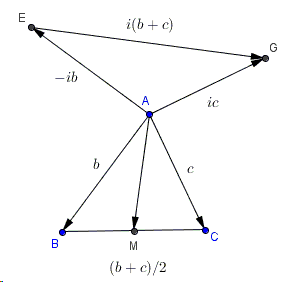# Two Properties of Flank Triangles - A Proof with Complex Numbers

Bottema's configuration of two squares that share a vertex is naturally embedded into Vecten's configuration of three squares erected on the sides of a triangle. The latter generalizes the first of Euclid's proofs of the Pythagorean theorem, so it rightfully refers to the Bride's Chair.

In the discussion of Vecten's configuration, we proved inter alia two properties of flank triangles that are a part of Bottema's configuration.1. If $AM$ is a median in $\triangle ABC$, $AH$ is an altitude in $\triangle AEG$, then $M$, $A$, $H$ are collinear.

2. $EG = 2\cdot AM$.

### ProofIn geometric problems, synthetic solutions are usually valued more than algebraic or trigonometric. However, complex numbers have a simple geometric meaning. In particular, multiplication by $i$ is equivalent to a counterclockwise rotation by $90^{\circ}$ whereas multiplication by $-i$ is equivalent to a clockwise rotation. Bearing this in mind, the proof appears to truly need no further explanation.

[Honsberger] credits the proof to Boris Pritsker of New York. It appeared in the now defunct journal Quantum (May/June 1996, p. 44).

### References

1. R. Honsberger, Mathematical Diamonds, MAA, 2003, 63-64### Properties of Flank Triangles